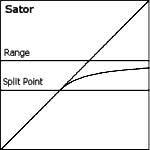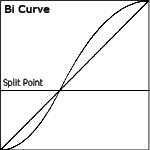soundbytes signal shapers

The Signal shaper Package includes:

• The Sator
• The Bi Curve
• A tiny electrical piano that demonstrates how these versatile tools can be used.

### Soundbytes Sator

A Shaper that executes a Saturation like compression on the signal Equaly useful as a

• waveshaper for amplitude modulation
• As Distortion Effect,
• for Midi controller scaling,
• or to compress a Signal.#### CONTROLS:

• [Range] - Sets the maximum output.
• [Split Pt] - The signal below the break point remaines untouched while Amplitudes above the threshold are compressed.
Note: The Split point position is relative to the Range parameter.
(SplitAmplitude = SplitPt * Range)

### Soundbytes Bi Curve

A Signal Shaper consisting of two parabolic parts. The lower portion has a negative curve while the upper curve is positive.
Very useful for Velocity or Midi Controller scaling. The curve can be blended into a straight line (x=y). Range(0 ... 1)#### CONTROLS:

• [Turn Pt] - Turning point. split point between lower and upper segment. (0.0001 ... 0.9999)
• [Lin/Curve] - Blends between linear and curved shape.
© andreas sumerauer 2012  contact information - info@soundbytes.de